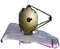# NIRISS FiltersJWST NIRISS has 12 medium- and broadband filters that cover the wavelength range between 0.8 and 5.0 μm in support of applications involving aperture masking interferometry, wide field slitless spectroscopy, and imaging.

On this page

Main article: NIRISS Observing Modes

NIRISS has a total of 12 filters, which are located in the NIRISS pupil and filter wheels as shown in Figure 1. The pupil wheel contains six short wavelength filters with central wavelengths between 0.9 and 2.0 μm, while the filter wheel has six long wavelength filters with central wavelengths between 2.8 and 4.8 μm. When used in combination with other optical elements, these filters support the NIRISS aperture masking interferometry (AMI), wide field slitless spectroscopy (WFSS), and imaging modes.Schematic diagram showing the location of bandpass filters in the NIRISS pupil (left) and filter (right) wheels. In the pupil wheel, short wavelength broad- and medium-band filters are indicated in blue and cyan, respectively. In the filter wheel, long wavelength broad- and medium-band filters are indicated in red and orange, respectively.

# Historical context

Except for F158M, the NIRISS filters originated as NIRCam "flight spares." Long wavelength filters that populate the NIRISS filter wheel were transferred directly. However, filters with central wavelengths shorter than 2.5 μm required the addition of a "low pass" filter to eliminate "red leaks" inherent in the design of their optical coatings.

These red leaks (from radiation at wavelengths >2.5 μm) did not affect NIRCam: the short wavelength filters were designed to be used with HgCdTe detectors with a 2.5 μm cut-off, which preclude the detection of photons at longer wavelengths. Since these photons were still detectable by the NIRISS detector (5.2 μm cutoff), the "double-stack" filter design shown in Figure 2 was implemented for all the filters in pupil wheel except F158M. Properties of the low pass filters are included in all estimates of the throughput of the short wavelength "double-stack" NIRISS filters.

As a result of their common heritage, the properties of the NIRISS filters are very similar to their counterparts in NIRCam, even when allowing for the addition of the "low pass" element for the short wavelength filters.Exploded view of the structure of a NIRISS "double-stack" filter. Source: Honeywell.

# Properties of the NIRISS filters

Main articles: NIRISS Sensitivity, NIRISS Bright Limits

Figure 3 illustrates the transmission curves for the entire suite of NIRISS filters. Key properties of the filters are quantified in Table 1.Transmission curves for the entire suite of NIRISS filters, based on measurements at cryogenic temperatures by the manufacturer.

Table 1. Properties of NIRISS filters

Filter

$\begin{array}{l}\lambda_{pivot}~(\mu\rm m)\,^1\end{array}$

BW (μm)2Effective response3

$\begin{array}{l}\lambda_{-} (\mu \rm m)\,^4\end{array}$

$\begin{array}{l}\lambda_{+} (\mu \rm m)^4\end{array}$

Observing mode
Broadband filters
F090W0.9000.1940.8800.7961.004WFSS, Imaging
F115W1.1500.2410.8391.0131.282WFSS, Imaging
F150W1.4980.3330.9361.3311.670WFSS, Imaging
F200W1.9840.4610.9451.7512.225WFSS, Imaging
F277W2.7760.7150.8752.4133.142AMI, Imaging
F356W3.5950.9210.9023.1414.068Imaging
F444W4.4351.1210.8583.8805.023Imaging
Medium-band filters
F140M1.4050.1470.8971.3321.480WFSS, Imaging
F158M1.5870.1960.8761.4881.688WFSS, Imaging
F380M3.8280.2050.8673.7263.931AMI, Imaging
F430M4.2860.2020.7944.1824.395AMI, Imaging
F480M4.8170.2980.6904.6694.971AMI, Imaging

1 The pivot wavelength, $\begin{array}{l}\lambda_{pivot}\end{array}$, relates the flux per unit wavelength ($\begin{array}{l}F_\lambda\end{array}$) to the flux per unit frequency ($\begin{array}{l}F_\nu\end{array}$). It is defined as:  $\begin{array}{l}\lambda^2_{pivot} = {\int{ \lambda T(\lambda)\, \rm{d}\lambda}}\,\, / \,\,{\int{ \left(T(\lambda)\,/\,\lambda \right)\, \rm{d}\lambda}}\end{array}$, where $\begin{array}{l}T(\lambda)\end{array}$ is the filter transmission curve.

2 The bandwidth BW is the integral of the filter transmission curve normalized by the maximum transmission ($\begin{array}{l}T_{max}\end{array}$):  $\begin{array}{l}BW = \int T(\lambda) \,{\rm d}\lambda\,\, /\,\, {T_{max}}\end{array}$.

3 The effective response is the mean transmission value over the wavelength range of $\begin{array}{l}\lambda_{pivot} \pm BW / 2\end{array}$.

4 The short ($\begin{array}{l}\lambda_{-}\end{array}$) and long ($\begin{array}{l}\lambda_{+}\end{array}$) half power wavelengths of a filter are the wavelengths at which its transmission falls to 50% of its peak value.

# NIRISS system throughput

Figure 4 shows the net photon-to-electron conversion efficiency (PCE) for each of the NIRISS filters.These values were estimated by combining current understanding of the throughput of the JWST Optical Telescope Element (OTE), the internal optical properties of NIRISS, and the quantum efficiency of the NIRISS detector. At any epoch, these effects are taken into account by the JWST Exposure Time Calculator (ETC). Although Figure 4 is useful for "back-of-the-envelope" calculations, critical applications should use the ETC to derive definitive estimates of performance.The figure shows net throughput curves for each of the NIRISS filters. Net throughput represents the estimated performance of the JWST optical telescope element and the internal optics of NIRISS.

# Ancillary data

NIRISS transmission and net throughput curves may be downloaded. Please see the accompanying "ReadMe" file for further information about this distribution.

Published 11 Jul 2017